## getting odetest to verify this Maple solution...

Any one has smart way to help verify this Maple solution to this ODE? I can't figure it out

```restart;
ode:=diff(y(x),x)-y(x) = x*y(x)^(1/2):
ic:= y(0)=4:
maple_sol:=dsolve([ode,ic],'implicit'):
the_diff_1:=odetest( (lhs-rhs)(maple_sol)=0,[ode,ic]);

maple_sol:=dsolve([ode,ic],'explicit'):
the_diff_2:=odetest( (lhs-rhs)(maple_sol)=0,[ode,ic]);
```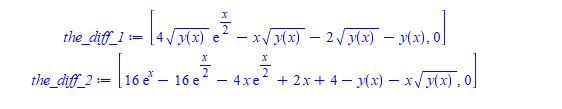Tried few simplifications but not everything. I am assuming Maple solution is correct.

Maple 2021.1

## Inconsistent(?) Dataframe indexing...

Background

This 'quirk' of Maple behaviour cropped up when I was considering solutions for the question posted here

https://www.mapleprimes.com/questions/232433-Extract-From-Record

where the OP had "poorly structured" data which meant it was a bit "awkward" to access required fields. A number of more-or-less satisfactory solution were proposed, and the OP seems happy

The Issue

One possible way to solve the original problem would be to restructure the data as a DataFrame. The "natural" way to do this would be to use numeric row indexes.. Note that these row indexes are not contiguous, and are in no particular order. However using this approach means that accessing fields from the dataframe is not consistent.

Sometimes the supplied row label is interpreted as a 'label', sometimes it is interpreted as the "row number". It appears that the latter interpretation is preferentially used

I can't make up my mind whether to call this a "bug" or not, but I can see the inconsistent interpretation resulting in chaos.

Before submitting an SCR I'd like to know if anyone else sees this as a bug

Consider the code

```  restart;
prettyprint=1:
#
# data
#
S := [`206` = Record(mu = 508.001018040,  sigma = 125.002863204708),
`4` = Record(mu = 1008.001018040, sigma = 167.707232430134),
`2` = Record(mu = 1208.001018040, sigma = 141.512246146314),
`5` = Record(mu = 808.001018040,  sigma = 117.156800098735)
]:
#
# Construct the above as a dataframe - note row labels are numbers!
#
DF:= DataFrame( Matrix([seq( [rhs(j):-mu, rhs(j):-sigma], j in S)]),
rows= [seq( parse( lhs(j) ), j in S)],
columns=[mu, sigma]
);
DF[206, mu];
DF[2, mu];  ## Errr No!
DF[5, sigma];
[           mu             sigma      ]
[                                     ]
[206  508.001018040   125.002863204708]
[                                     ]
DF := [ 4   1008.001018040  167.707232430134]
[                                     ]
[ 2   1208.001018040  141.512246146314]
[                                     ]
[ 5   808.001018040   117.156800098735]

508.001018040

1008.001018040

117.156800098735

```

Note that DF[2, mu] outputs the entry from the second row - not the one from the row labelled with the number 2

I imagina a similar issue would occur with numeric column indexes, although I haven't tried this

## How do I setup solve to find only the real and ima...

restart:
with(plots):
kernelopts(version);

How do I setup solve to find only the real and imaginary part of Zeta to be both positive and the real part of Zeta to be the smallest positive value? For example: real part = 1.348412872 and imaginary part = 0.04230725448.

Acceleration ratio (db) and phase (deg). Convert ratio to linear and phase to radian.
dat := <14.52407334|-162.1310124>:
A := 10^(dat/20.):
phi := dat*Pi/180.:
R:= 0.3036:
Characteristic equation Eqn 5
f := (Zeta,A,phi) -> cos(Zeta) - R * Zeta * sin(Zeta) - exp(-phi * I)/A:

soln := [solve(f(Zeta,A,phi), Zeta)]:

Tests.mw

## how to obtain this implicit solution for this ODE?...

Textbook gives this nice short implicit solution for this ode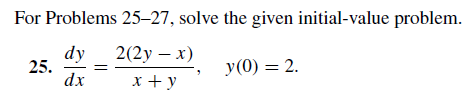As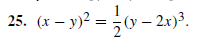But Maple does not give an implicit solution

```restart;
ode:=diff(y(x),x) = 2*(2*y(x)-x)/(x+y(x));
ic:=y(0)=2;
sol:=dsolve([ode,ic],'implicit')
```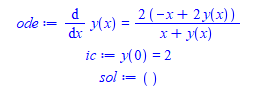Removing implicit gives very complicated solution as it tries to solve for y(x).

```book_sol:=(x-y(x))^2-1/2*(y(x)-2*x)^3=0;
odetest(book_sol,[ode,ic])```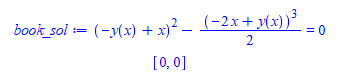Any suggestion or a trick or a different approach to make Maple generate the same solution given in the textbook?

ps. manually, it is possible to obtain the same solution as book as follows

```restart;
ode:=diff(y(x),x) = 2*(2*y(x)-x)/(x+y(x));
ic:=y(0)=2;
sol:=dsolve(ode,'implicit');
sol:=simplify(exp(lhs(sol))=exp(rhs(sol)));
the_constant:=solve(sol,_C1);
the_constant:=eval(the_constant,[y(x)=2,x=0]);
sol:=eval(sol,_C1=the_constant);
odetest((lhs-rhs)(sol)=0,[ode,ic])

```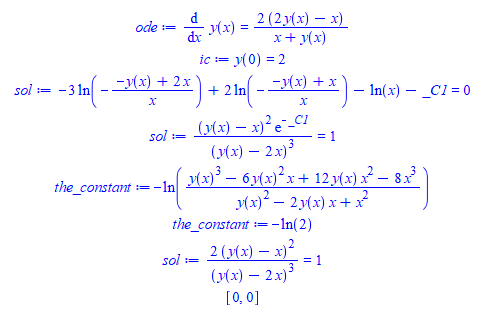edit june 14,2021

I found a direct way to get an implicit solution which is close enough to book solution. It is by using Lie symmetry method

```restart;
ode:=diff(y(x),x) = 2*(2*y(x)-x)/(x+y(x));
ic:=y(0)=2;
sol:=dsolve([ode,ic], y(x),'implicit','Lie');
```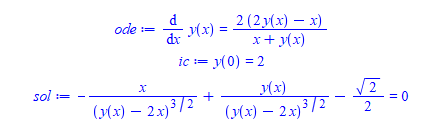Another variant which gives book solution but requires one extra step

```restart;
ode:=diff(y(x),x) = 2*(2*y(x)-x)/(x+y(x));
ic:=y(0)=2;
sol:=dsolve([ode,ic], y(x),'implicit','Lie',way=all,fat);
simplify(exp( (lhs-rhs)(sol)))=1

```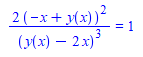ps. corrected above now, thanks to comment below by Carl.

Maple 2021.1

## why command completion popup behave different when...

In worksheet mode, when typing only the first 3 letters of the command piecewise, the command completion popup shows and I can hit TAB to have it automatically complete which is nice since I always makes typos tying this command

>pie

When when I wanted to get help on the same command, I have to type 5 letters now before the popup shows to help me complete the command

>?piece

Why is that? I would have expected the same 3 letters to be enough, as I assume Maple is smart enough to notice this.

Here is a movie to illustrate. This is Maple 2021.1 on windows 10## an efficient way to remove objects from list, if o...

I have a list of objects. Each object contains many fields, one of these fields happened to be a solution to an ode.

At the end of solving the ODE, I'd like to remove all objects which contain the same solution.

I can not do the normal trick in Maple to remove duplicates from a list, which is to convert the list to a set and let Maple automatically remove duplicates because in this case each entry in the list is an object of many fields and hence each object is always different.

I only want to remove the objects from the list which has the same specific field.

Currently what I do is the following:   Do a first scan, and loop over all entries in the list to find the duplicates. Each time I find a duplicate solution, mark a field in the object as it is being duplicate.

Then do another scan at the end, to build a new list, which only adds those objects not marked as duplicates.

It works, but was wondering if Maple or someone might have better suggestion or more elegent way to do this.

In this MWE I am using a list and adding objects to it making it grow dynamically. I know it would be better to use Array, but for now I'd like to stick to a list, since the number of solutions is normally small. May be in the future I will change the list to Array to avoid building a list dynamically.

This example code builds a list of 5 objects

```restart;
ode_solution_type:=module()
option object;
export sol:=NULL; #only ONE solution here.
export stuff;
export mark_to_delete:=false;
end module:

SOL::list:=[]:  #where to keep the objects

for n from 1 to 5 do
tmp:=Object(ode_solution_type);

if n=3 then
tmp:-sol:=y(x)=1;
else
tmp:-sol:=y(x)=0;
fi;
tmp:-stuff:=rand();
SOL:=[op(SOL),tmp];
od:
```

In the above, I made 4 objects to have same solution which is y(x)=0 and one different. The goal is to remove all objects from the list which has duplicate solutions and keep only one copy.

```for n from 1 to numelems(SOL) do
print("sol ", n , "  is ", SOL[n]:-sol);
od:
```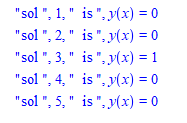And this is how currently I remove the duplicates

```for n from 1 to numelems(SOL) do
for m from n+1 to numelems(SOL) do
if is(SOL[n]:-sol=SOL[m]:-sol) and not SOL[m]:-mark_to_delete then
print("found duplicate at ",m);
SOL[m]:-mark_to_delete:=true;
fi;
od;
od:

#now make new pass to keep the non- duplicates
new_SOL::list:=[]:
for n from 1 to numelems(SOL) do
if not SOL[n]:-mark_to_delete then
new_SOL:=[op(new_SOL),SOL[n]];
fi;
od:
```

checking:

```for n from 1 to numelems(new_SOL) do
print("sol ", n , "  is ", new_SOL[n]:-sol);
od:
```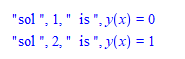Does there exist a better option in Maple (while still using a list?) to remove objects in list which have the same specific field?

Could you suggest a better method?

## Can one obtain infinity as a solution for a variab...

sometimes I get a solution to an ODE, where when I plugin the initial conditions to solve for the constant of integration, the solution by inspection can be seen to be infinity.

The constant of integration is allowed to be infinity.

But now I get no solution from solve and so my program rejects this solution and says the ode can't be solved because it can't find value for the constant of integration using this initial conditions.

I am not sure how to handle this, since Maple solve will not return an infinity as possible solution for the constant of integration. I tried different assumptions.

This has to work for general, and not for this specific example I will show now.

Here is an ODE with IC

```ode:=diff(y(x),x)=y(x)^3*sin(x);
ic:=y(0)=0;
sol:=[dsolve(ode)];```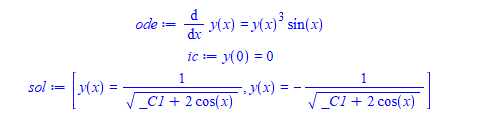The general solution is easy to find since this is just separable. The problem is when I plugin the initial conditions. (again, this is done in a program, without looking at the screen). let consider the first solution above for now.

`eq:=simplify(subs( [y(x)=0,x=0],sol));`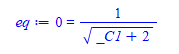And here is the problem, when next I try to solve for the constant, Maple says no solution.

`solve(eq,_C1,allsolutions=true) assuming _C1::real`

No solution returned.

If Maple could return _C1=infinity, then I would plugin _C1=infinity and obtain the correct particular solution which is

`simplify(subs(_C1=infinity,sol)) assuming real`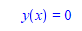Which is what the smart dsolve command does

```ode:=diff(y(x),x)=y(x)^3*sin(x);
ic:=y(0)=0;
sol:=dsolve([ode,ic])```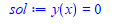I do not know how Maple found the above and what algorithm it used.

But my not too smart dsolve program gets stuck on such problems, because it can't solve for the IC.

Is there another method or option or assumptions to use, to solve such an equation and request infinity be returned as a solution?  I tried isolate(eq,_C1) but that did not work.

One possible option to try as a last resource is to do this

`limit(eq,_C1=infinity)`

And this gives 0=0

which means _C1=infinity is valid solution.  And I could do this as final attempt before giving up. Is this what Maple did? But how does one know to do this in general? Unless one tries and find out? i.e I could try limit(eq,_C1=infinity) and limit(eq,_C1=-infinity) and see if any one of these gives true (after doing  evalb or is  on the output)

Any suggestions or a better/more robust method to use for such cases? I need to use the method for any equation where infinity can be a solution for the constant of integration. It will always be one equation and one variable, and can assume all are real for now if it makes it easier.

## why dsolve do not show this solution?...

I was wondering why dsolve do not show this one solution I obtained different way, for this ode.

Maple gives 6 solutions to the ODE. But 5 of them are signular (have no constant of integration in them). I am looking at the last solution it gives, the one with constant of integration.

But when I solve this ODE, after simplifying it, I get different general solution than the last one Maple shows. I thought they might be equivalent, but I do not see how they could be.

So my question is why Maple did not show the simpler solution also?  Here is the code

`ode_orginal:=1/3*(-2*x^(5/2)+3*y(x)^(5/3))/x^(3/2)*diff(y(x),x)/y(x)^(5/3)+1/2*(2*x^(5/2)-3*y(x)^(5/3))/x^(5/2)/y(x)^(2/3) = 0;`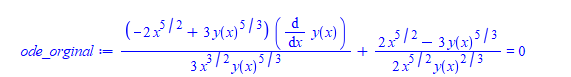This looks complicated and non-linear, but when I solve for y'(x) it gives much simpler and linear ODE (Have to convert the ODE to D before solving for diff(y(x),x), since Maple can complain otherwise).

```ode:=convert(ode_orginal,D);
ode:=diff(y(x),x)=solve(ode,D(y)(x),allsolutions=true);
```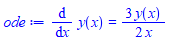And solving the above gives the much simpler solution

```sol:=dsolve(ode,y(x));
odetest(sol,ode);```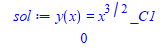Now when using dsolve on the original complicated lookin ODE it gives

```maple_sol:=[dsolve(ode_orginal)];
```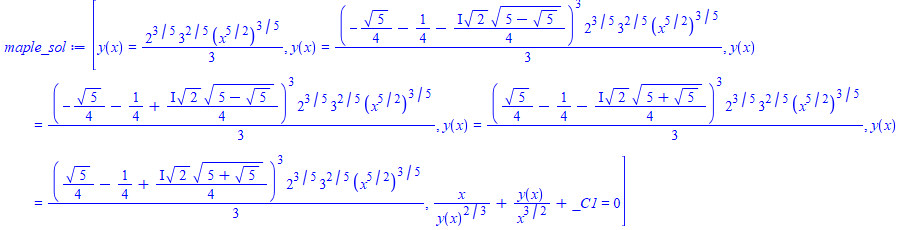if there is any hope that one of the above 6 solutions will be the same as the simpler solution, it has to be the last one, with the _C1 in it, since all the others do not have _C1 (singular solutions).

But solving for y(x) from the last one does not give the simpler solution.

So it is a new general solution for the original ode and it is correct, since odetest gives zero.

I think in the process of solving for y'(x), some solutions got lost. Even though I asked for allsolutions there?.

But my main question is: Should dsolve have also have given the simpler solution? Since that one also satisfies the original nonlinear complicated ode

```odetest(sol,ode_orginal);
```

gives 0.

Maple 2021.1

## why odetest does not verify this solution against ...

I solved this ode with IC, and obtained a solution.  Maple odetest says my solution satisfies the ODE but not the initial conditions. But I do not see why. When I plugin manually the initial conditions into the solution, I get true.

So I am not sure what is going on.

```restart;
ode:=x*diff(y(x),x)*y(x) = (x+1)*(y(x)+1);
ic:=y(1)=1;
mysol:=y(x)-ln(y(x)+1)=-ln(2)+x+ln(x);
my_sol_at_IC:=subs([y(x)=1,x=1],mysol);

#now check manually
evalb(my_sol_at_IC);
is(my_sol_at_IC);
```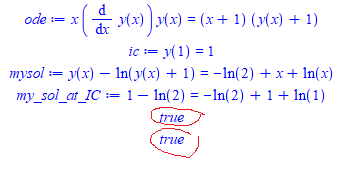Using odetest gives

```odetest(mysol,ode);
odetest(mysol,[ode,ic]);
```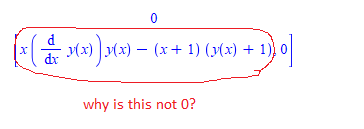What Am I doing wrong?

Maple 2021.1

Edit June 12, 2021

This is another example where odetest gives different result depending on how the solution is written !

```restart;
ode:=diff(y(x),x)=2/3*(y(x)-1)^(1/2):
mysol_1:=y(x) = 1/9*x^2 - 2/9*x + 10/9:
mysol_2:=y(x) - (1/9*x^2 - 2/9*x + 10/9) = 0 :
odetest(mysol_1,ode);
odetest(mysol_2,ode);
```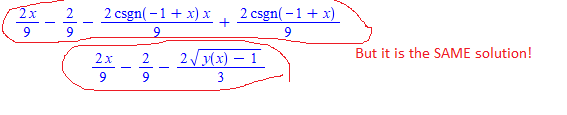## strange result using evalb() in debugger vs. outsi...

I can't figure what is going on here. I have an expression, where when I run the program, I see inside the debugger that the result of evalb(simplify(tmp_1)) where tmp_1 is the expression, gives false

When I copy the same tmp_1 expression to new worksheet and do evalb(simplify(tmp_1)) it gives true.

I added print statements in the code to print tmp_1 and print result of simplify(tmp_1) and for some reason, it gives false when I run the program, but it gives true when I do the same thing in a work sheet, by copying tmp_1 and trying it there.

Never seen anything like this, as expression is all numbers. Here is the print out when I run the program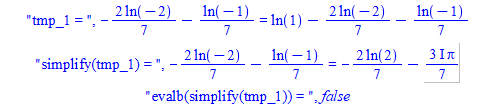Here is the same thing in worksheet

```restart;
tmp_1 :=-(2*ln(-2))/7 - ln(-1)/7 = ln(1) - (2*ln(-2))/7 - ln(-1)/7;
simplify(tmp_1);
evalb(simplify(tmp_1));
```

true

and if you think I made mistake somewhere, here is also screen shot from the debugger window itself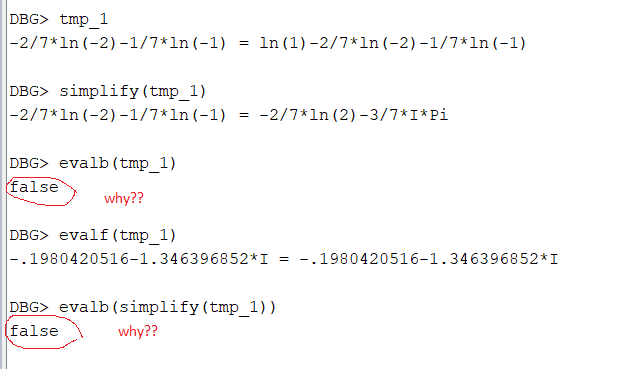again, same thing gives true in separate worksheet, copying same exact expression from above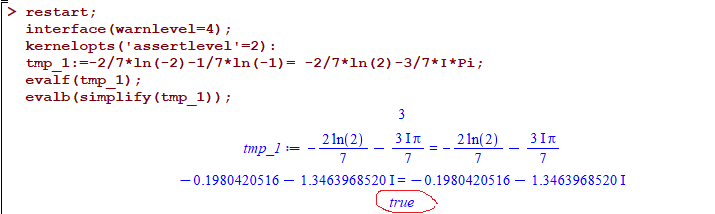There seem to be something loaded when I run my program that causes this difference, but I have no idea now what it is.  This has nothing to do with the debugger loaded btw, I am just using the debugger to show the details. When I run the program, without the debugger, I get false for the above, and I was trying to find why.

Any suggestions what to try and what to check for and what could cause this? Should it not give true when I run the program also? Could it be some Digits setting changed when the program run? I do not change any system defaults of any sort when running the program.

Maple 2020.1

edit june 12, 2021

Here is another manifestation of the same problem I just found. There is something seriously wrong for it to behave this way.

This is an expression called tmp_1 in a local variable in a proc inside a module. There are not even any local symbols in it. When I run the program, all the attempts to show it is true fail. Some give FAIL and some give false when the result should be true

When I simply copy the expression from the debugger window to an open worksheet, I get true

Here is the screen shot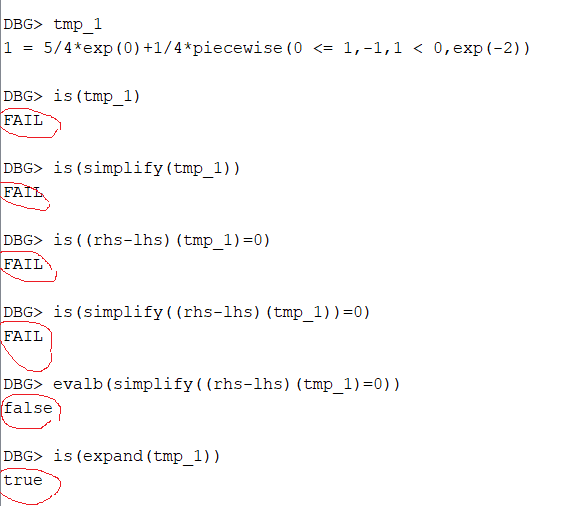Only when I did is(expand(tmp_1)) did it give true

But when I copy the expression to the worksheet, it gives true using all the other attempts:

```restart;
tmp_1:=1 = 5/4*exp(0)+1/4*piecewise(0 <= 1,-1,1 < 0,exp(-2)):
is(tmp_1);
is(simplify(tmp_1));
is((rhs-lhs)(tmp_1)=0);
is(simplify((rhs-lhs)(tmp_1)=0));
evalb(simplify((rhs-lhs)(tmp_1)=0));
is(expand(tmp_1))
```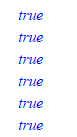I can't make a MWE so far, since these problems only show up with I run my large program. I think Maple internal memory get messed up or something else is loaded that causes these stranges problems.

For now, I added expand() to the things I should try. My current code now looks like

```if is(tmp_1) or is(simplify(tmp_1)) or is((rhs-lhs)(tmp_1)=0)
or is(simplify((rhs-lhs)(tmp_1)=0)) or evalb(simplify((rhs-lhs)(tmp_1)=0))
or is(expand(tmp_1)) then
....
```

Maple 2021.1

## Two remarks about discrete distributions in Maple...

by: Maple 2021

... and two suggestions to the development team

POINT 1
In ?DiscreteValueMap (package Statistics) it's given an example concerning rhe Geometric distribution along with this comment:
"The Geometric distribution is discrete but it necessarily assumes integer values, so (bold font is mine) it also does not have a DiscreteValueMap"

This sentence seems to indicate that "because a distribution is discrete over the set of integers, it cannot have a DiscreteValueMap", some sort of logical implication...

But my feeling is that the Geometric distribution (or any other discrete distribution) does not have a DiscreteValueMap because this attribute has just not been specified when defining the distribution.

```restart:
with(Statistics):

GeomRV := RandomVariable(Geometric(1/2)):
f := unapply(ProbabilityFunction(GeomRV, n), n):

AnotherGeomRV := Distribution(
'ProbabilityFunction'=f,
'Support'=0..infinity,
'DiscreteValueMap'=(n->n),
'Type'=discrete
):
DiscreteValueMap(AnotherGeomRV , n);
```

Thus having the set of natural numbers as support doesn't imply that DiscreteValueMap cannot exist.

Suggestion 1: modify the ?DiscreteValueMap help page so that it no longer suggests that some discrete distributions cannot have a .DiscreteValueMap

______________________________________________________________________________________

POINT 2
I think there exists a true problem with the definition of discrete distributions in Maple: the ProbabilityFunction of a (discrete) random variable) takes non zero values outside their definition set.
For instance

`ProbabilityFunction(GeomRV, Pi);  # something non null`

To ivercome this problem I defined a new Geometric distribution this way (not entirely satisfying):

```restart:
with(Statistics):

GeomRV := RandomVariable(Geometric(1/2)):
f := unapply(ProbabilityFunction(GeomRV, n), n):
g := n -> (1-ceil(n-floor(n)))*f(n)    # (1-ceil(n-floor(n))) = 1 if n in Z, 0 otherwise

AnotherGeomRV := Distribution(
'ProbabilityFunction'=g,
'Support'=0..infinity,
'DiscreteValueMap'=(n->n),  # is wanted
'Type'=discrete
):
ProbabilityFunction(AnotherGeomRV, 2);
1/8
ProbabilityFunction(AnotherGeomRV, Pi);
0
```

PS: None of the statistics based upon the  ProbabilityFunction (Mean, Variance, ... ) is correctly computed with the previous construction. This could be easily overcome by completing this definition, just as its done in Maple, for all the requires statistics, for instance

```AnotherGeomRV := Distribution(
....
'Mean'=1   # or more generally (1-p)/p form Geometric(p)
):```

Suggestion 2: modify the way discrete distributions are defined in Maple in order to avoid ProbabilityFunction to return wrong values.

## how to change subexpression with some specific pat...

I need to change any occurance of   anything*sqrt(anything)  to say Z in any large expression.  (later, I can add the correct replacement once I know how to do it for Z).

I can change   sqrt(anything) with the help of the answers in Substitution-Of-Sub-Expressions-Is  but not  anything*sqrt(anything)

Here is an example to make it clear. Given

`expr:=(-x + sqrt(9*x^2*exp(2*c) + 8)*exp(-c)+99)/(4*x)+ (a*sqrt(z)-99+sin(c*sqrt(r+4)+20))/3+10+1/(c+exp(-x)*sqrt(exp(x)))+sqrt(h)`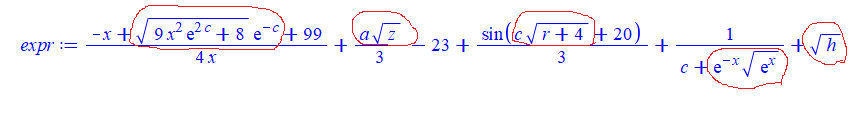Need to all some transformation on  those subexpressions circled above. For now, lets say I wanted to replace them with so it should becomes this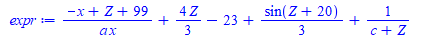I can do this

`subsindets(expr,anything^({1/2,-1/2}),ee->Z)`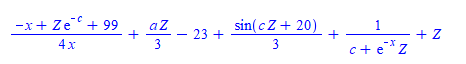But I need to have the term (if any) that multiplies the sqrt as well included.

And that is the problem. Can't figure how to do it. When I try

`subsindets(expr,anything*anything^({1/2,-1/2}),ee->Z)`

Maple says Error, testing against an invalid type Ok. So anything*anything^({1/2,-1/2}) is not a type. What to do then?  Tried also subsindets(expr,t::anything*anything^({1/2,-1/2}),ee->Z) same error

And

```applyrule(t1::anything*sqrt(t0::anything)=Z, expr);
```

gives  which is wrong.

How to make this work in Maple using subsindets? Can one use pattern with subsindets? How to make a type for

anything*sqrt(anything)

Maple 2021.1

## How to suppress axes labels...

I want to plot a 2D graph without labels. The labes=["",""] option does half of the job—it prints the empty string for labels (that's good) but it reserves room for them (that's bad).  In the following code I use a large size labelfont in order to exaggerate the effect:

```restart;
plots:-setoptions(labelfont=[TIMES,64]);  # large labelfont selected on purpose
p1 := plot([[0,0],[1,1]], labels=["", ""]);```Note the large blank space at the bottom reserved for the the non-existent label.

I know one way to eliminate the label altogether:

`p2 := subs(AXESLABELS=NULL, p1);`This does the right job but is there a more orthodox way of doing that?

Afterthought:  It would be good if the labels option to the plot command  accepted none as argument, as in labels=[none, none].

## on output format from solve with exponential...

Why Maple likes to extract exp() outside the sqrt when its argument has minus sign vs. not?  Compare the following

```restart;
eq2 := ln(2*u^2 + u - 1) = -c - 2*ln(x);
sol:=[solve(eq2,u)]:
simplify(sol)
```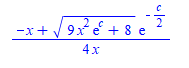and

```eq2 := ln(2*u^2 + u - 1) = c - 2*ln(x);
sol:=[solve(eq2,u)]:
simplify(sol)
```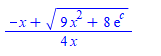I like the above much better than the first one. Mathematica keeps both same form (i.e. keeps the exp() inside):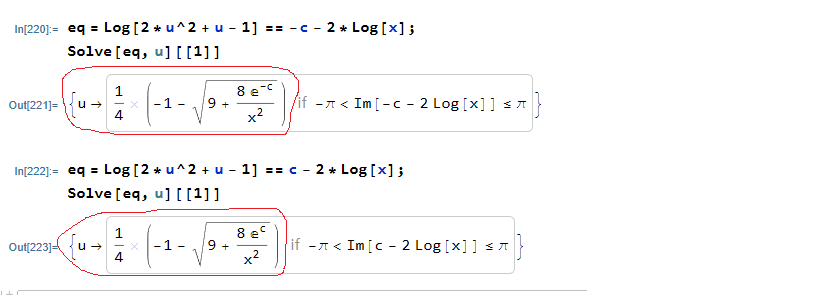Maple's answers are correct ofcourse, I just do not understand the logic why when there is a minus sign on it likes to format it differently as shown.

Is there a way to make not do that?

## Exporting 3D Plot in Vector Graphics Format...

I am trying to export a 3D plot from Maple (2021) in a vector format that can be imported into Adobe Illustrator without too much trouble. The graphic artist says the PDF files are not useful (“at the base of every PDF is an image file, inside layers and layers of clipping paths; not helpful”). “SVG does not appear to produce usable vectors – well, maybe not, but the one you sent me was super difficult to wrangle”. She had hoped that EPS would be useful, but she was unable to open the EPS file I created (not enough memory).

Here is the example that I have been using as I've asked others for help with this question.

```restart;
with(plots);
with(plottools);

T := torus([0, 0, 0], 1/2, 1/2);
C0 := spacecurve([cos(t), sin(t), 0], t = 0 .. 2*Pi, color = red, thickness = 9);
C1 := spacecurve([0.5*cos(t), 0.5*sin(t), 0.5], t = 0 .. 2*Pi, color = red, thickness = 9);
C2 := spacecurve([0.5*(1 + cos(t)), 0, 0.5*sin(t)], t = 0 .. Pi, color = blue, thickness = 9);
C3 := spacecurve([0.5*(-1 + cos(t)), 0, 0.5*sin(t)], t = 0 .. Pi, color = blue, thickness = 9);
Fig9510 := display([T, C0, C1, C2], view = [DEFAULT, DEFAULT, 0 .. 1/2], scaling = constrained, transparency = 0.75);```

Direct links to the worksheet and the PDF, EPS, and SVG files on DropBox are provide below in the hope that something will be useful to somebody. (MaplePrimes links are provided for the MW and PDF files are also provided - but EPS and SVG files are not permitted.)

1. 3DPlotExample.mw: https://www.dropbox.com/s/mx1hg1ntuqihnu3/3DPlotExample.mw?dl=0
2. Fig9510.pdf: https://www.dropbox.com/s/bnzfqrh060gw84g/Fig9510.pdf?dl=0
3. Fig9510.eps: https://www.dropbox.com/s/h9bjyn0uwm8w5ct/Fig9510.eps?dl=0
4. Fig9510.svg: https://www.dropbox.com/s/u2xfntnqvbhu810/Fig9510.svg?dl=0

This question has been asked for more than ten years. The best solution to has been to use the command-line-interface version of Maple.

1. Is the command-line interface still the best/only option for vector-based graphics output?
2. If so, how do i access the command-line version of Maple 2021 on MacOS?
﻿